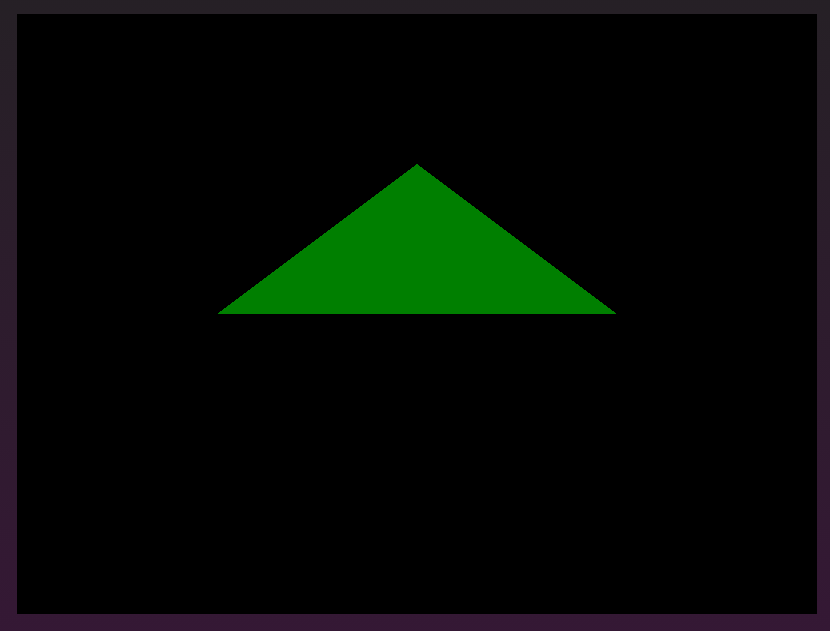﻿ WebGPU uniform颜色-多媒体-IT技术博客

# 简介# 1 创建 `uniform buffer`

``````const uniformBufferSize = 4 * 4 // vec4<f32>，即 16 byte
const uniformBuffer = device.createBuffer({
size: uniformBufferSize,
usage: GPUBufferUsage.UNIFORM | GPUBufferUsage.COPY_DST,
})
/* 创建绑定组对象，细节参考上篇 */
const uniformBindGroup = device.createBindGroup({
layout: bindGroupLayout, // <- 直接传递 绑定组布局对象，它是什么请参考上一篇
entries: [
{
binding: 0,
resource: { // <- 指定资源类型为 buffer，并传入 GPUBuffer 类型的对象
buffer: uniformBuffer
}
}
]
})

/* 创建类型数组，并写入 GPUBuffer */
const uniformColor = new Float32Array([0.0, 0.5, 0.0, 1.0])
device.queue.writeBuffer(uniformBuffer, 0, uniformColor.buffer, uniformColor.byteOffset, uniformColor.byteLength)
``````

# 2 修改着色器

## 顶点着色器

``````[[builtin(position)]] var<out> out_position: vec4<f32>;
[[location(0)]] var<in> in_position_2d: vec2<f32>;

[[stage(vertex)]]
fn main() -> void {
out_position = vec4<f32>(in_position_2d, 0.0, 1.0);
return;
}
``````

## 片元着色器

``````// 声明一个结构体类型，其包含一个我们需要的颜色字段
[[block]] struct Uniforms {
uniform_color: vec4<f32>;
};

// 声明一个结构体变量，将其绑定到 js 代码中绑定组的第 0 个资源值
[[binding(0), group(0)]] var<uniform> uniforms: Uniforms;
[[location(0)]] var<out> outColor: vec4<f32>;

[[stage(fragment)]]
fn main() -> void {
outColor = uniforms.uniform_color; // <- 从 uniform 里取一个颜色值
return;
}
``````

END